## TN State Board 11th Computer Science Important Questions Chapter 7 Composition and Decomposition

Question 1.
What is Pseudo code?
Pseudo code is inbetween English and high level computer languages. In English, the sentences may be long and may not be precise. In computer languages, the syntax has to be followed meticulously. If these two irritants are removed then we have the Pseudo code.

Question 2.
What is flow chart?

1. Flowchart is a diagrammatic notation for representing algorithms.
2. A flowchart is a collection of boxes containing statements and conditions which are connected by arrows showing the order in which the boxes are to be executed.Question 3.
Write the advantages of flow chart.

1. They are precise. They represent our thoughts exactly.
2. It is easy to understand small flow charts.

Question 4.
Write the disadvantages of flow chart.

1. Flowcharts are less compact than representation of algorithms in programming language or pseudo code.
2. They obscure the basic hierarchical structure of the algorithms.
3. Alternative statements and loops are disciplined control flow structures. Flowcharts do not restrict us to disciplined control flow structures.

Question 5.
What are the three important control flow statements.
There are three important control flow statements:

1. Sequential
2. Alternative
3. Iterative.Question 6.
Define sequential statement.
A sequential statement is composed in the sequence are executed one after another, in the same order as they are written in the algorithm, and the control flow is said to be sequential. Let SI and S2 be statements. A sequential statement composed of S1 and S2 is written as

S1
S2

Question 7.
Define compound statement.
Statements may be composed of other statements, leading to a hierarchical structure of algorithms. Statements composed of other statements are known as compound statements.

Question 8.
Give the notations for algorithms.
We need a notation to represent algorithms. There are mainly three different notations for representing algorithms.

1. A programming language is a notation for expressing algorithms to be executed by computers.
2. Pseudo code is a notation similar to programming languages. Algorithms expressed in pseudo-code are not intended to be executed by computers, but for communication among people.
3. Flowchart is a diagrammatic notation for representing algorithms. They give a visual intuition of the flow of control, when the algorithm is executed.Question 9.
Write short notes on variant of alternative statement.
Some times we need to execute a statement only if a condition is true and do nothing if the condition is false. This is equivalent to the alternative statement in which the else- clause is empty. This variant of alternative statement is called a conditional statement. If C is a condition and S is a statement, then
if C
S
is a statement, called a conditional statement, that describes the following action:
(i) Test whether C is true or false.
(ii) If C is true then do S; otherwise do nothing.
The conditional control flow, is depicted in the flowchart of Figure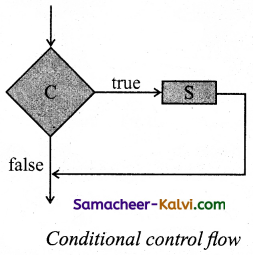Question 10.
Explain the process of iterative statement.
An iterative process executes the same action repeatedly, subject to a condition C. If C is a condition and S is a statement, then while C
S
is a statement, called an iterative statement, that describes the following action:
(i) Test whether C is true or false.
(ii) If C is true, then do S and go back to step 1; otherwise do nothing.

The iterative statement is commonly known as a loop. These two steps, testing C and executing S, are repeated until C becomes false. When C becomes false, the loop ends and the control flows to the statement next to the iterative statement. The condition C and the statement S are called the loop condition and the loop body, respectively. Testing the loop condition and executing the loop body once is called an iteration. Now C is known as the termination condition.

Iterative control flow is depicted in the flowchart of Figure. Condition C has two outgoing arrows, true and false. The true arrow points to S box. If C is true, S box is executed and control flows back to C box. The false arrow points to the box after the iterative statement (dotted box). If C is false, the loop ends and the control flows to the next box after the loop.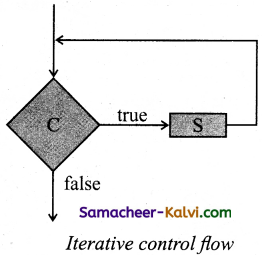Question 11.
Write an algorithm to compare two numbers and produces the result as
compare(a, b) = {-1 if a < b, 0 if a = b, 1 if a > b}
1. case a < b
2. result :=-1
3. case a = b
4. result := 0
5. else– a > b
6. result: = 1

Question 12.
Describe the action of the alternative statement with an example.
A condition is a phrase that describes a test of the state. If C is a condition and both S1 and S2 are statements, then
if C
S1
else
S2
is a statement, called an alternative statement, that describes the following action:
(i) Test whether C is true or false.
(ii) If C is true, then do S1; otherwise do S2.

In pseudo code, the two alternatives S1 and S2 are indicated by indenting them from the keywords if and else, respectively. Alternative control flow is depicted in the flowchart of Figure. Condition C has two outgoing arrows, labeled true and false. The true arrow points to the SI box. The false arrow points to S2 box. outgoing arrows of SI and S2 point to the same box, the box after the alternative statement.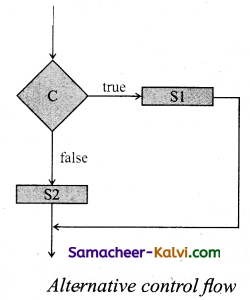Question 13.
Write the specification and algorithm to find minimum of two numbers.
The specification of algorithm minimum is
— minimum(a, b)
— input s : a , b
— outputs: result = a↓b
Algorithm minimum can be defined as
1. minimum(a, b)
2. — a, b
3. if a < b
4. result: = a
5. else
6. result = b
7. — result = a↓b

Question 14.
Write the characteristics of algorithm.
A well defined algorithm has the five basic characteristic as follows.
(i) Input:
Algorithm starts with procedural steps to accept input data. The algorithm must accept one or more data to be processed.

(ii) Definite:
Each operational step or operation must be definite (i.e.) each and every instruction must clearly specify that what should be done.

(iii) Effective: Each operational step can atleast in principle is carried out by a person using a paper and pencil in a . minimum number of times.

(iv)Terminate:
After some minimum number of operation algorithm must come to an end.

(v) Output:
An algorithm is written to solve the problem, therefore it must produce one or more computed.Question 15.
Draw a flow chart to estimate the volume of a box using its length, breadth and height.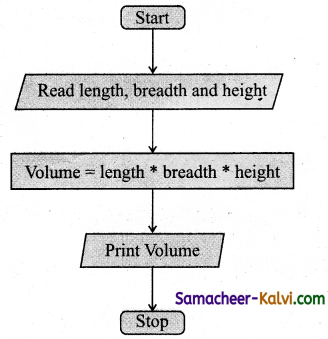Question 16.
The state changes for the goat, grass and wolf problem as solved in Example 6.2 (Textbook Page No. 117) are shown in Figure 7.13. The values of farmer, goat, grass and wolf are denoted by -4tuples such as LLLL. Write a sequence of state changes is possible from LLLL to RRRR. Find it.
1. – farmer, goat, grass, wolf = L, L, L, L
2. farmer, goat := R, R
3. — farmer, goat, grass, wolf = R, R, L, L
4. farmer := L
5. farmer, goat, grass, wolf = L, R, L, L
6. farmer, grass := R, R
7. — farmer, goat, grass, wolf = R, R, R, L
8. farmer, goat := L, L
9. — farmer, goat, grass, wolf = L, L, R, L
10. farmer, wolf := R, R
11. — farmer, goat, grass, wolf = R, L, R, R
12. farmer: = L
13. – farmer, goat, grass, wolf = L, L, R, R
14. farmer, goat: = R, R
15. — farmer, goat, grass, wolf = R, R, R, RQuestion 17.
Draw a flow chart for integer division.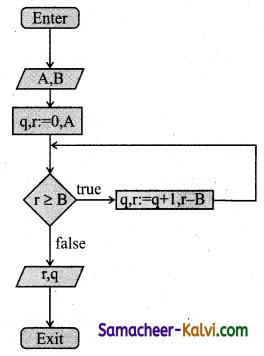Question 18.
Draw the different types of boxes used in the flow chart. Explain each one of its roles.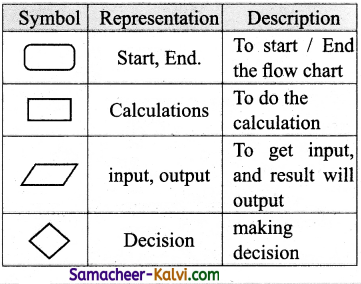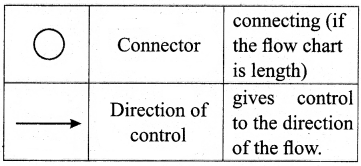Question 19.
On the island of chrome land there are three different types of chameleons: red chameleons, green chameleons and blue chameleons whenever two chameleons of different colours meet they both change color to the third colour. In the end, all should display the same colour.
Let us represent the number of chameleons of each type by variables a, b and c, and their initial values by A, B and C, respectively. Let a – b be the input property. The input-output relation is a = b = 0 and c = A+B+C. Let us name the algorithm monochromatize. The algorithm can be specified as
monochromatize(a, b, c)
– inputs: a=A, b=B, c=C, a=b
— outputs: a = b = 0 , c = A+B+C
In each iterative step, two chameleons of the two types (equal in number) meet and change, their colors to the third one. Eg: if A, B, C = 4, 4, 6, then the series of meetings will result in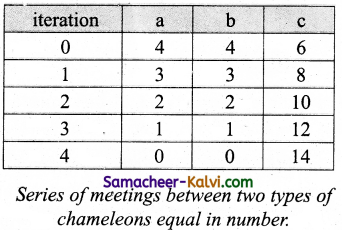In each meeting, a and b each decreases by 1 and c increases by 2. The solution can be expressed as an iterative algorithm. monochromatize(a, b, c)
— inputs: a=A, b=B, c=C, a=b
— outputs: a = b = 0, c = A + B + C
while a > 0
a, b, c:= a – 1; b – 1; c + 2
The algorithm is depicted in the flowchart of Figure.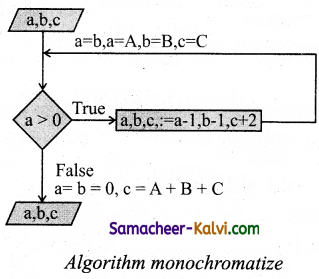Question 20.
Draw a flow chart to eat breakfast.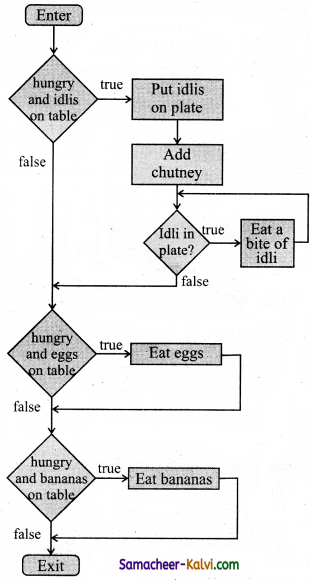Question 21.
Using function minimum(a, b) define a function minimum 3(a, b, c) to output the minimum of three numbers a, b, and c.
The specification of an algorithm minimum is minimum (a, b, c)
— inputs : (a, b, c)
— outputs: a↓b↓c
Algorithm minimum can be defined as
1. minimum (a, b, c)
2. -a, b, c
3. x = a
4. if b < x then
5. x = b
6. else
7. if c < x then
8. x = c
9. x = a↓b↓ c.

Question 22.
Draw a flow chart to read a number between 0 and 3 and write it in words.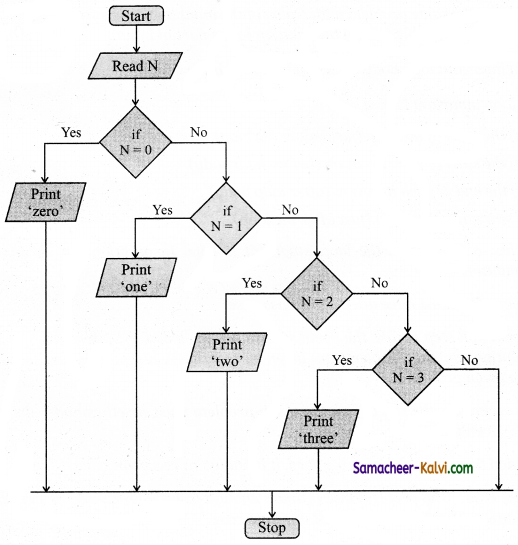Question 23.
Distinguish between a condition and a statement.

 Condition Statement The conditions are specified by a set of conditional statements having the boolean expressions which are evaluated to a boolean value true or false. A statement is a command given to the computer that instructs the computer to take a specific action. A computer program is made up of a series of statements.

Question 24.
Draw a flowchart for conditional statement.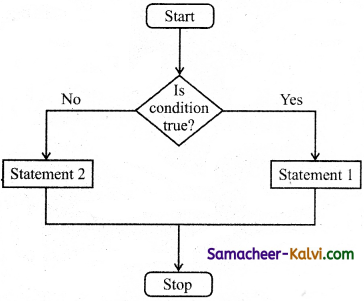Question 25.
Both conditional statement and iterative statement have a condition and a statement. How do they differ?
The conditional statement is executed only if the condition is true. Otherwise, nothing is done. Whereas the iterative statement repeatedly evaluates a condition and executes a statement as long as the condition is true.Question 26.
What is the difference between an algorithm and a program?

 Algorithm Program An algorithm is a list of steps, typically written in some human language, that explains how to perform a specific task. A program is a list of instructions that tells a computer exactly what to do from step to step.

Question 27.
Why is function an abstraction?
After an algorithmic problem is decomposed into subproblems, we can abstract the j subproblems as functions. A function is like a sub-algorithm. Similar to an algorithm, a j function is specified by the input property and the desired input-output relation.

Question 28.
How do we refine a statement?
After decomposing a problem into smaller subproblems, the next step is either to refine the subproblem or to abstract the subproblem.

1. Each subproblem can be expanded into more detailed steps. Each step can be further expanded to still finer steps, and so on. This is known as refinement.
2. We can also abstract the subproblem. We specify each subproblem by its input property and the input-output relation, While solving the main problem, we only need to know the specification of the subproblems. We do not need know how the subproblems are solved.Question 29.
For the two flowcharts given below. Write the pseudo code.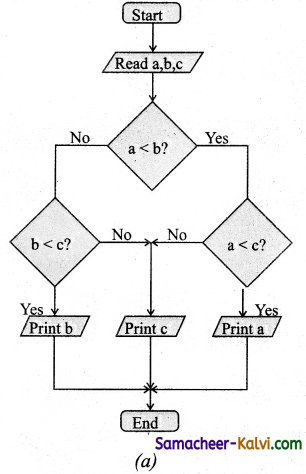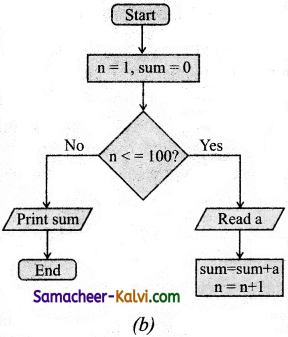(a) start
if a < b then
(if a < c then
print a
else
print c
)
else
(if b < c then
print b
else
print c)
end.

(b) start
sum = 0
n = 1
while n <= 100 then do
sum = sum + a
n = n + 1
print sum
end.Question 30.
If C is false in line 2, trace the control flow in this algorithm.
1 S1
2 — C is false
3 if C
4 S2
5 else
6 S3
7 S4
(i) Test the condition C is false.
(ii) If C is false the statement SI, S3, S4 is executed.

Question 31.
What is case analysis?
Case analysis splits the problem into an exhaustive set of disjoint cases. For each case, the problem is solved independently. If Cl, C2 and C3 are conditions and S1, S2, S3 and S4 are statements, a 4-case analysis statement has the form,
1. case C1
2. S1
3. case C2
4. S2
5. case C3
6. S3
7. else
8. S4

The conditions C1, C2, and C3 are evaluated in turn. For the first condition that evaluates to true, the corresponding statement is executed, and the case analysis statement ends. If none of the conditions evaluates to true, then the default case S4 is executed.
(i) The cases are exhaustive: at least one of the cases is true. If all conditions are false, the default case is true.
(ii) The cases are disjoint: only one of the cases is true. Though it is possible for more than one condition to be true, the case analysis always executes only one case, the first one that is true. If the three conditions are disjoint, then the four cases are (1) C1, (2) C2, (3) C3, (4) (not C1) and (not C2) and (not C3).Question 32.
Draw a flowchart for -3case analysis using alternative statements.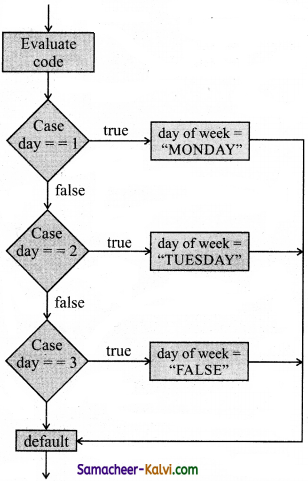Question 33.
Define a function to double a number in two different ways: (1) n + n, (2) 2 × n.
(1) double (n)
— inputs : n
— outputs : n + n
(2) double (n)
— inputs : n
— outputs: 2 × nQuestion 34.
Exchange the contents: Given two glasses marked A and B. Glass A is full of apple drink and glass B is full of grape drink. Write the specification for exchanging the contents of glasses A and B, and write a sequence of assignments to satisfy the specification.
drinks (A, B)
— inputs: A, B where A is Apple drink, B is grape drink
— outputs: A (grape drink), B (apple drink)
such that
t: = A
A : = B
B : = t

Question 35.
Circulate the contents: Write the specification and construct an algorithm to circulate the contents of the variables A, B and C as shown below: The arrows indicate that B gets the value of A, C gets the value of B and A gets the value of C.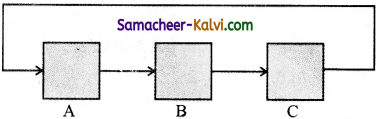The specification of the algorithm for circulating the contecontents (a, b, c)
— inputs : a = 10, b = 20, C = 30 -outputs : a = 30, b = 10, C = 20 Algorithm for circulating the contents 0 start
2. temp = b
3. b = a
4. a = c
5. c = temp
6. print a, b, c
7. End

Question 36.
Decanting problem. You are given three bottles of capacities 5, 8 and 3 litres. The 8L bottle is filled with oil, while the other two are empty. Divide the oil in 8L bottle into two equal quantities. Represent the state of the process by appropriate variables. What are the initial and final states of the process? Model the decanting of oil from one bottle to another by assignment. Write a sequence of assignments to achieve the final state.
The goal is to construct a statement so as to move from initial state to final state.
Let A = 5 litre, B = 8 litre, C = 3 litre
— outputs: A (grape drink), B (apple drink) such that
1. A, B, C = 0, 8; 0 (initial state)
2. S
3. A, B, C = 4, 4, 0
Now we have to write a sequence of assignment statement to achieve the final statement.
1. –A, B, C = 0, 8, 0 (initial state)
2. –A, B: = 3, 5
(pour 3 litre in the first bottle)
3. –A, B, C = 3, 5, 0
4. B, C: = 3/2
(pour 2 litre from bottle B to C)
5. –A, B, C = 3, 3, 2
6. –A, B := 6, 0
(pour 3 litre from bottle B to A)
7. –A, B, C = 6, 0, 2
8. A, B:= 1, 5
(pour 5 litre from bottle A to B)
9. –A, B, C = 1, 5, 2
10. B, C = 4, 3
(pour 1 litre from bottle B to C)
11. —A, B, C = 1, 4, 3
12. A, C := 4, 4
(pour three litre from bottle C to A)
13. –A, B, C = 4, 4, 0Question 37.
Trace the step-by-step execution of the algorithm for fartorial(4). factorial(n)
— inputs : n is an integer, n > 0
— outputs:f = n!
f, i = 1, 1
while i < n
f, i := f × i, i + 1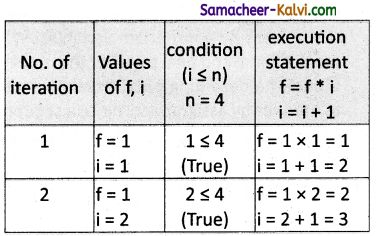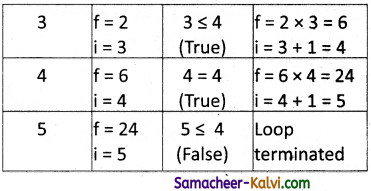Factorial (4) = 24
(i) Read the value n = 4
(ii) Assign the value of f = 1, i = 1
(iii) Check the condition i < n if it is true the statement f = f * i
i = i + 1 is executed.
(iv) When the condition goes false the control variable i comes out of the loop and print the result.
Output: n = 24Question 1.
(a) sequence to print data
(b) selection
(c) repetition
(d) all of above
(d) all of above

Question 2.
__________ is a diagrammatic notation for representing algorithms.
(a) Psuedo code
(b) Flow chart
(c) Statement
(d) Diagrams
(b) Flow chart

Question 3.
The rectangle shaped symbol is used to show the:
(a) errors
(b) decision
(c) process
(d) input / output
(c) processQuestion 4.
________ is a mix of programming language – like constructs and plain English.
(a) Pseudo code
(b) Algorithm
(c) Flow chart
(d) Program
(a) Pseudo code

Question 5.
There are ________ important control flow statements.
(a) 2
(b) 3
(c) 4
(d) 5
(b) 3

Question 6.
The ________ statements in the sequence are executed one after another.
(a) sequential
(b) alternative
(c) iterative
(d) control
(a) sequentialQuestion 7.
The variant of alternative statement is called a _______ statement.
(a) sequential
(b) control
(c) conditional
(d) Iterative
(c) conditional

Question 8.
An ________ process executes the same action repeatedly.
(a) iterative
(b) non-iterative
(c) flow charts
(d) pseudo code
(a) iterative

Question 9.
The elementary problem – solving techniques is:
(a) composition
(b) iteration
(c) decomposition
(d) refinement
(c) decompositionQuestion 10.
A _______ is like a sub-algorithm.
(a) Function
(b) Arrays
(c) Pseudo code
(d) Structure
(a) Function

Question 11.
_________ represents a comparison, that determinates alternative paths to be followed:

(a)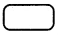(b)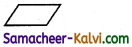(c)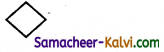(d)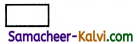(c)Question 12.
Statements composed of other statements are known as _______ statements.
(a) simple
(b) compound
(c) sequential
(d) iterative
(b) compoundQuestion 13.
Connector is represented by a:
(a) rectangle
(b) square
(c) oval
(d) small circle
(d) small circle

Question 14.
Control flow statements are called:
(a) compound statements
(b) looping statements
(c) branching statements
(d) control statements
(a) compound statements

Question 15.
A sub problem can be expanded to finer steps:
(a) Decomposition
(b) Refinement
(c) Abstract
(d) Decompose
(b) RefinementQuestion 16.
Which is used as a function in solving other problems?
(a) Black box
(b) Red box
(c) Square box
(d) Blue box
(a) Black box

Question 17.
Identify the incorrect statement:
(a) Pseudo-code is a mix of programming – language like constructs and plain English.
(b) Flowchart is a diagrammatic notation for representing algorithms.
(c) A statement is a phrase that commands the computer to do an action.
(d) A sequential statement is not composed of a sequence of statements.
(d) A sequential statement is not composed of a sequence of statements.

Question 18.
Choose the odd from the control flow statements:
(a) Sequential
(b) pseudo-code
(c) Alternative
(d) Iterative
(b) pseudo-codeQuestion 19.
Match the following: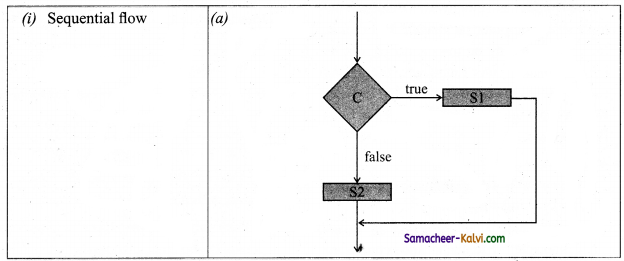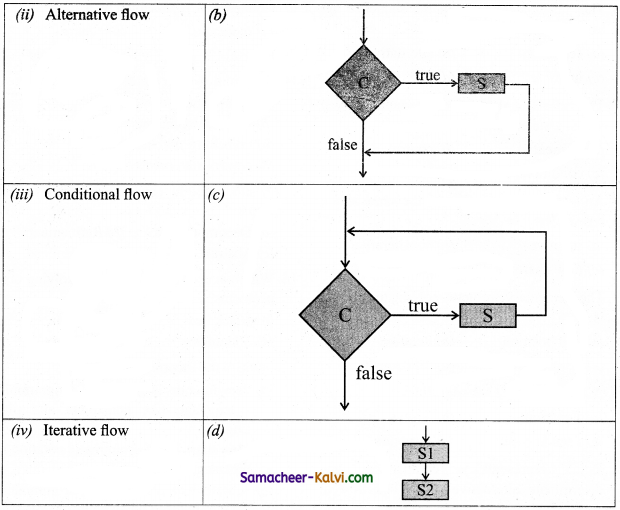(a) (i) – (a); (ii) – (b); (iii) – (d); (iv) – (c)
(b) (i) – (d); (ii) – (a); (iii) – (b); (iv) – (c)
(c) (i) – (b); (ii) – (c); (iii) – (a); (iv) – (d)
(d) (i) – (b); (ii) – (d); (iii) – (c); (iv) – (a)
(b) (i) – (d); (ii) – (a); (iii) – (b); (iv) – (c)

Question 20.
Suppose u, v = 10, 5 before the assignment. What are the values of u and v after the sequence of assignments?
1 u := v
2 v := u
(a) u, v = 5, 5
(b) u, v = 5, 10
(c) u, v = 10, 5
(d) u, v=10,10
(a) u, v = 5, 5Question 21.
Which of the following properties is true after the assignment (at line 3?
1– i + j = 0
2 i, j := i + 1, j – 1
3– ?
(a) i + j > 0
(b) i + j < 0
(c) i + j = 0
(d) i = j
(c) i + j = 0

Question 22.
If C1 is false and C2 is true, the compound statement:
1 if C1
2 SI
3 else
4 if C2
5 S2
6 else
7 S3
executes
(a) S1
(b) S2
(c) S3
(d) none
(b) S2Question 23.
If C is false just before the loop, the control flows through:
1 S1
2 while C
3 S2
4 S3
(a) S1 ; S3
(b) S1 ; S2 ; S3
(c) S1 ; S2 ; S2 ; S3
(d) S1 ; S2 ; S2 ; S2 ; S3
(a) S1 ; S3

Question 24.
If C is true, SI is executed in both the flowcharts, but S2 is executed in: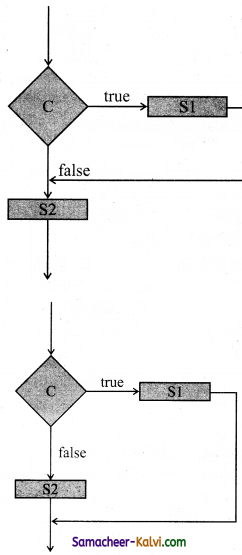(a) (1) only
(b) (2) only
(c) both (1) and (2)
(d) neither (1) nor (2)
(a) (1) onlyQuestion 25.
How many times the loop is iterated?
i := 0
while i ≠ 5
i := i + 1
(a) 4
(b) 5
(c) 6
(d) 0